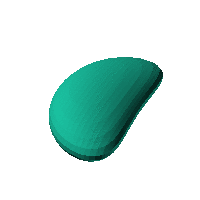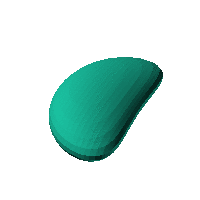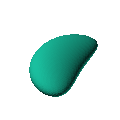Blob Farm# blob30The blob in all its glory:Nice crescent by inverting a ellipsoid on a sphere.

 Click on the snapshot to download the blob's stl file.Octave Code:
```  # name of the blob
project = "blob30";

function w = f(x2,y2,z2,c,r,e)
x  = (x2-c(1))/r(1);
y  = (y2-c(2))/r(2);
z  = (z2-c(3))/r(3);
# function at origin must be <0, and >0 far enough away.  w=0 defines the surface

w=x.^2+y.^2+z.^2 - 1.0;

endfunction;

# this is for distorting the grid before applying the function
# note that the undistorted grid will be used to make the stl file
# just set it to x3=x; y3=y; z3=z; if no warping is needed.
function [x3,y3,z3]= prewarp(x,y,z)
R =200;  # center of sphere is at (R+X0,0,0) with radius R (passing through (X0,0,0) )
X0 = 0; # this means that parts of the blob near [X0,0,0] will stay near that point.
# calculate the inverted coordinate of each point in the 3D grid (x3,y3,z3)
x2 = x-R-X0;  y2=y;   z2 = z; # intermediate values
r2 = R*R./(x2.^2+y2.^2+z2.^2);
x3 = x2.*r2+R+X0;  y3 = y2.*r2;  z3 = z2.*r2;
endfunction;

c_outer = [0,0,0];
r_outer = [35,75,20];

source("../octave/func2stl_v01.m");  # do all the calculations
```
GNU Octave